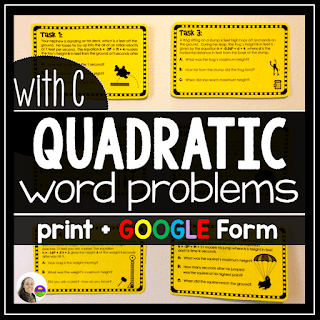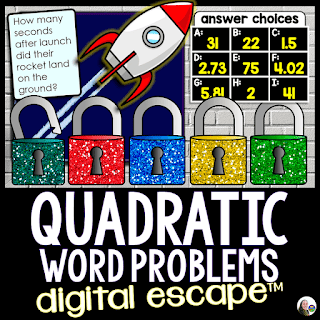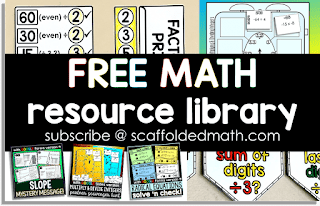## Pages

"But Miss, these word problems don't have any numbers!"

"You're right! They're numberless word problems!"

This was the conversation with my algebra 2 students at the start of our quadratic word problems unit every year. I LOVE teaching quadratics, especially because students always start super intimidated and end the unit super confident in themselves for having accomplished something big!

But the language! How long, how high, hits the ground, time in the air... these simple sounding phrases can be pretty confusing at first. To focus on what these phrases meant and what they were asking us to find, I liked to start our projectile motion unit with a numberless quadratics activity. Presenting students with word problems with no numbers forced students to look for the quadratic keywords as clues to what they were being asked to find.

Here's an example of a numberless quadratics problem:

"Jack wants to know how high the soccer ball will get if he kicks it straight up in the air."

How high is code for y at the vertex, so students would need to identify that this question is asking them for the y coordinate at the vertex.

Here's another question:

"How do I find when an object hits the ground?"

For this question, students need to identify the way(s) to find when an object hits the ground in a quadratic word problem. We can either use the Zero function on our graphing calculators or use the Quadratic Formula to find the positive zero of the parabola.

Identifying the keywords in quadratic word problems was always the hardest part of this unit for my students. Once they understood what a problem was asking them to find, the solving was fairly easy.

s a day-to-day reminder of the language they'd see in quadratic word problems,  made my students a big 4-page poster out of this Quadratic Keywords poster

You can find the numberless quadratics activity we used in class here in my Dropbox: numberless quadratics activityQuadratic Word Problems Task CardsQuadratic Word Problems Digital Math Escape RoomFree math resource library